## Example Questions

### Example Question #10 : Right Triangles

The ratio for the side lengths of a right triangle is 3:4:5. If the perimeter is 48, what is the area of the triangle?

96

108

50

48

240

96

Explanation:

We can model the side lengths of the triangle as 3x, 4x, and 5x. We know that perimeter is 3x+4x+5x=48, which implies that x=4. This tells us that the legs of the right triangle are 3x=12 and 4x=16, therefore the area is A=1/2 bh=(1/2)(12)(16)=96.

### Example Question #61 : Plane Geometry

The length of one leg of an equilateral triangle is 6. What is the area of the triangle?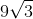Explanation: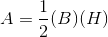The base is equal to 6.

The height of an quilateral triangle is equal to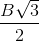, whereis the length of the base.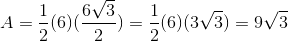### Example Question #62 : Plane Geometry

Find the area of the following right triangle to the nearest integer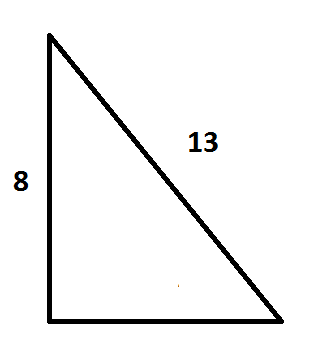Note: The triangle is not necessarily to scale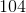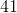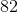Explanation:

The equation used to find the area of a right triangle is: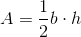where A is the area, b is the base, and h is the height of the triangle. In this question, we are given the height, so we need to figure out the base in order to find the area. Since we know both the height and hypotenuse of the triangle, the quickest way to finding the base is using the pythagorean theorem,. a = the height, b = the base, and c = the hypotenuse.

Using the given information, we can write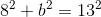. Solving for b, we get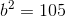or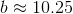. Now that we have both the base and height, we can solve the original equation for the area of the triangle.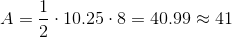### Example Question #63 : Plane Geometry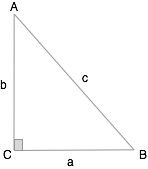In the above triangle, if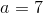and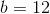what is the area of the triangle?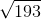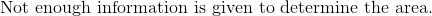Explanation:

To find the area of a triangle use the formula: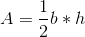, since the base isand the height is, plugging in yields:### Example Question #71 : Plane Geometry

A right triangle has integer sides with a ratio of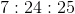, measured in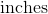. What is the smallest possible area of this triangle?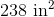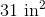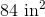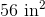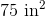Explanation:

The easiest way to find the smallest possible integer sides is to simply factor the ratio we are given. In this case,is already prime (sinceis a prime number), so the smallest possible sides which hold to this triangle are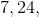and. You may also recognize this number as a common Pythagorean triple.

The area of a triangle is expressed as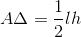, whereis the length andis the height. Since our triangle is right, we know that two lines intersect at aangle and thus serve well as our length and height. We also know that the longest side is always the hypotenuse, so the other two sides must beand.

Applying our formula, we get: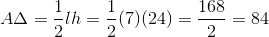Thus, the smallest possible area for our triangle is.

### Example Question #72 : Plane Geometry

Right triangle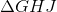has hypotenuse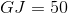cm and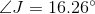. Find the area of the triangle, in cm2, by using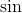.

Round angles to four significant figures. Round side lengths to the nearest integer.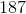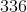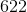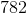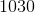Explanation:

To find the area of a right triangle, find the lengths of the two perpendicular legs (since this gives us our length and height for the area formula).

In this case, we know that one angle is, and SOHCAHTOA tells us that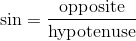, so: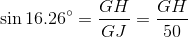Substitute the angle measure and hypotenuse into the formula.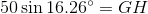Isolate the variable.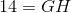Solve the left side (rounding to the nearest integer) using our Pythagorean formula:--->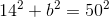Substitute known values.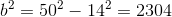--->  Simplify.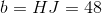Square root both sides.

So with our two legs solved for, we now only need to apply the area formula for triangles to get our answer: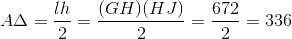So, the area of our triangle is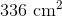.

### Example Question #73 : Plane Geometry

Find the area of a right triangle whose height is 4 and base is 5.Explanation:

To solve, simply use the formula for the area of a triangle given height h and base B.

Substitute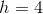and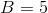into the area formula.

Thus,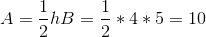### Example Question #1 : How To Find The Area Of A Right Triangle

A right triangle has a total perimeter of 12, and the length of its hypotenuse is 5. What is the area of this triangle?

3

10

15

6

12

6

Explanation:

The area of a triangle is denoted by the equation 1/2 b x h.

b stands for the length of the base, and h stands for the height.

Here we are told that the perimeter (total length of all three sides) is 12, and the hypotenuse (the side that is neither the height nor the base) is 5 units long.

So, 12-5 = 7 for the total perimeter of the base and height.

7 does not divide cleanly by two, but it does break down into 3 and 4,

and 1/2 (3x4) yields 6.

Another way to solve this would be if you recall your rules for right triangles, one of the very basic ones is the 3,4,5 triangle, which is exactly what we have here